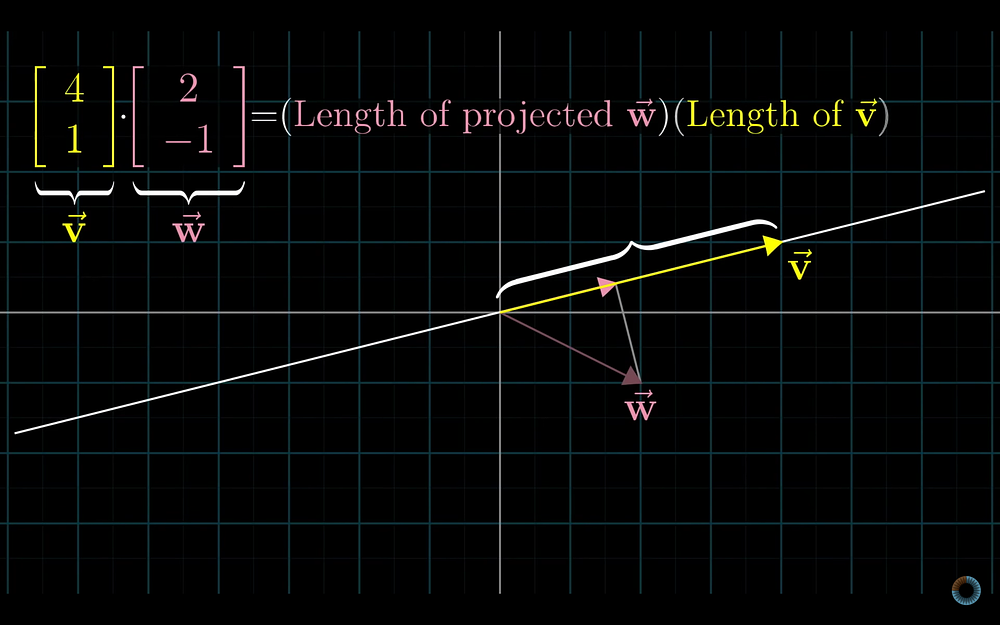# Linear Algebra Explained — Part 3But why is this calculation related with the idea of projection? To understand that, let’s take a look at linear transformations from multiple dimensions to one dimension. They are like functions taking multi dimensional vectors and outputting one dimensional vectors, which is just numbers. In part 1, it is explained that transformations are called linear when the grid lines remain parallel and evenly spaced. Similarly, for linear transformations to one dimension, if we select evenly spaced dots, they remain evenly spaced.

We also saw in part 1 that transformations are defined with the coordinates that basis vectors land, in this case i^and j^. In the case where the transformation is from two dimensions to one dimension, corresponding matrix is 1×2.

To apply this transformation to a vector, we multiply a matrix of 1×2 by a 2D vector. This calculation is same as dot product.

Therefore, we can say that there is an association between 1×2 matrices and 2D vectors. But what does this association mean geometrically?

To understand the geometric meaning of the association between 1×2 matrices and 2D vectors, let’s imagine that we put a number line on top of 2D coordinate space diagonally and the number zero sitting at the origin. Let’s also define a 2D vector u^whose tip sits where the number one on the number line. After that, let’s define a projection transformation. This transformation is linear because evenly spaced dots remain evenly spaced after the transformation.

Since the transformation is linear, we can represent it as a 1×2 matrix. We need to find where i^and j^lands and those numbers will be the columns of the matrix.

Since u^, i^ and j^have unit length, we can use symmetry here. Following images explain how i^lands on ux and j^lands on uy. Therefore, entries of the matrix is the coordinates of u^.